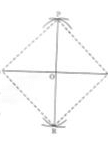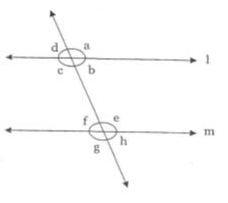# Test: Constructions- 1

## 25 Questions MCQ Test Online MCQ Tests for Class 9 | Test: Constructions- 1

Description
Attempt Test: Constructions- 1 | 25 questions in 25 minutes | Mock test for Class 9 preparation | Free important questions MCQ to study Online MCQ Tests for Class 9 for Class 9 Exam | Download free PDF with solutions
QUESTION: 1

Solution:
QUESTION: 2

Solution:
QUESTION: 3

### On a ray AB with initial point A, Taking A as centre and some radius, draw an arc of a circle, which intersects AB, say at a point D. Taking D as centre and with the same radius as before, draw an arc intersecting the previously drawn arc, say at a point E. Draw the ray AC passing through E. Then, the measure of ∠CAB is

Solution:
QUESTION: 4

It is not possible to construct a triangle whose sides are

Solution:
QUESTION: 5

A rotating ray after making a complete rotation coincides with its initial position. The angle thus formed is

Solution:
QUESTION: 6

With the help of a rular and compass, it is not possible to construct an angle of

Solution:
QUESTION: 7

If two circles touches internally then distance between their centres is equal to

Solution:
QUESTION: 8

If a 600 angle is bisected twice what will be measure of each angle that is constructed ?

Solution:
QUESTION: 9

It is not possible to construct a triangle ABC with BC = 5cm,∠B = 75and AB+AC  equal to

Solution:
QUESTION: 10

If two lines are parallel, the perpendicular distance between the

Solution:
QUESTION: 11

The construction of △ABC in which AB = 5 cm, ∠A = 750 is not possible when difference of BC and AC is equal to

Solution:
QUESTION: 12

Radius of a circle is ________ to tangent

Solution:
QUESTION: 13

In the following below PR is the perpendicular bisector of a line segment AB = 16 cm, then which of the following is true ?Solution:
QUESTION: 14

With the help of a ruler and a compass, it is possible to construct an angle of

Solution:
QUESTION: 15

How many angles are formed by a transversal with a pair of lines?Solution:
QUESTION: 16

The construction of △ABC, given that BC = 5 cm, ∠B = 600 is not possible when the difference of AB and AC is equal to

Solution:
QUESTION: 17

Two radii of the same circle are always:

Solution:

Two Radii and a chord make an isosceles triangle. The perpendicular from the centre of a circle to a chord will always bisect the chord (split it into two equal lengths). Angles formed from two points on the circumference are equal to other angles, in the same arc, formed from those two points.parallel and may inchired at any angle.

QUESTION: 18

An external bisector of an angle measuring 700 will divide the angle into two angles measuring

Solution:
QUESTION: 19

The construction of a triangle ABC with AB = 4 cm and ∠A = 600 is not possible when difference of BC and AC is equal to

Solution:
QUESTION: 20

How many pairs of corresponding angles are formed when a transversal intersects a pair of lines?

Solution:
QUESTION: 21

The construction of a triangle ABC, given that BC = 3 cm, ∠C = 600 is possible when the difference of AB and AC is equal to

Solution:
QUESTION: 22

A quadrilateral can be drawn.If the measures of its

Solution:
QUESTION: 23

In △ ABC, which of the following information is needed to construct it if it is known that measure of ∠B = 60 and BC = 6 cm :

Solution:
QUESTION: 24

The construction of a triangle ABC with AB = 6 cm and ∠A = 600 is not possible when sum of BC and CA is equal to

Solution:
QUESTION: 25

How many pairs of alternate angles are formed when a transversal intersects a pair of lines?

Solution:Use Code STAYHOME200 and get INR 200 additional OFF Use Coupon Code